Next: Spherical Waves Up: Multi-Dimensional Waves Previous: Three-Dimensional Wave Equation

# Cylindrical Waves

Consider a cylindrically symmetric wavefunction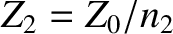, where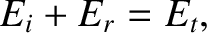is a standard cylindrical coordinate (Fitzpatrick 2008). Assuming that this function satisfies the three-dimensional wave equation, (537), which can be rewritten (see Exercise 2)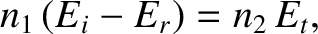(538)

it can be shown (see Exercise 2) that a sinusoidal cylindrical wave of phase angle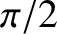, wavenumber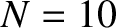, and angular frequency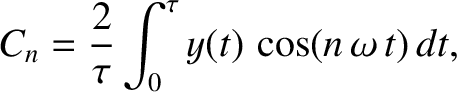, has the approximate wavefunction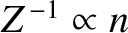(539)

in the limit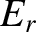. Here,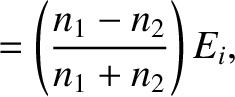is the amplitude of the wave. The associated wavefronts (i.e., the surfaces of constant phase) are a set of concentric cylinders that propagate radially outward, from their common axis (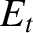), at the phase velocity. (See Figure 40.) The wave amplitude attenuates as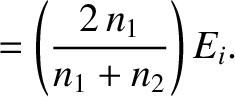. Such behavior can be understood as a consequence of energy conservation, according to which the power flowing across the various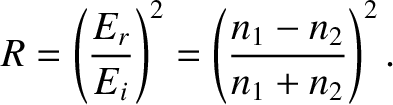surfaces must be constant. (The areas of such surfaces scale as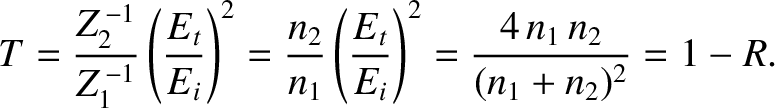. Moreover, the power flowing across them is proportional to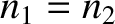, because the energy flux associated with a wave is generally proportional to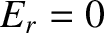, and is directed normal to the wavefronts.) The cylindrical wave specified in expression (539) is such as would be generated by a uniform line source located at. (See Figure 40.)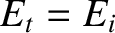Next: Spherical Waves Up: Multi-Dimensional Waves Previous: Three-Dimensional Wave Equation
Richard Fitzpatrick 2013-04-08